Related Articles
Boolean Indexing in Pandas
• Difficulty Level : Medium
• Last Updated : 13 Jan, 2021

In boolean indexing, we will select subsets of data based on the actual values of the data in the DataFrame and not on their row/column labels or integer locations. In boolean indexing, we use a boolean vector to filter the data.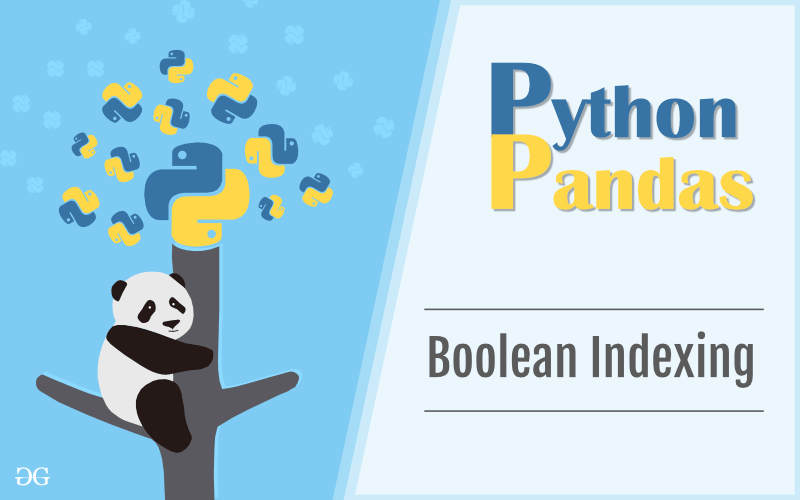Boolean indexing is a type of indexing which uses actual values of the data in the DataFrame. In boolean indexing, we can filter a data in four ways –

• Accessing a DataFrame with a boolean index
• Applying a boolean mask to a dataframe
• Masking data based on column value
• Masking data based on index value

Accessing a DataFrame with a boolean index :
In order to access a dataframe with a boolean index, we have to create a dataframe in which index of dataframe contains a boolean value that is “True” or “False”. For Example

## Python3

 `# importing pandas as pd` `import` `pandas as pd` ` `  `# dictionary of lists` `dict` `=` `{``'name'``:[``"aparna"``, ``"pankaj"``, ``"sudhir"``, ``"Geeku"``],` `        ``'degree'``: [``"MBA"``, ``"BCA"``, ``"M.Tech"``, ``"MBA"``],` `        ``'score'``:[``90``, ``40``, ``80``, ``98``]}` ` `  `df ``=` `pd.DataFrame(``dict``, index ``=` `[``True``, ``False``, ``True``, ``False``])` ` `  `print``(df)`

Output: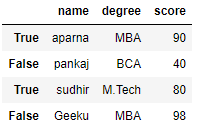Now we have created a dataframe with boolean index after that user can access a dataframe with the help of boolean index. User can access a dataframe using three functions that is .loc[], .iloc[], .ix[]

#### Accessing a Dataframe with a boolean index using .loc[]

In order to access a dataframe with a boolean index using .loc[], we simply pass a boolean value (True or False) in a .loc[] function.

## Python3

 `# importing pandas as pd` `import` `pandas as pd` ` `  `# dictionary of lists` `dict` `=` `{``'name'``:[``"aparna"``, ``"pankaj"``, ``"sudhir"``, ``"Geeku"``],` `        ``'degree'``: [``"MBA"``, ``"BCA"``, ``"M.Tech"``, ``"MBA"``],` `        ``'score'``:[``90``, ``40``, ``80``, ``98``]}`   `# creating a dataframe with boolean index ` `df ``=` `pd.DataFrame(``dict``, index ``=` `[``True``, ``False``, ``True``, ``False``])`   `# accessing a dataframe using .loc[] function ` `print``(df.loc[``True``])`

Output: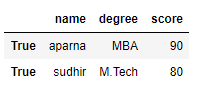#### Accessing a Dataframe with a boolean index using .iloc[]

In order to access a dataframe using .iloc[], we have to pass a boolean value (True or False)  but iloc[] function accept only integer as argument so it will throw an error so we can only access a dataframe when we pass a integer in iloc[] function
Code #1:

## Python3

 `# importing pandas as pd` `import` `pandas as pd` ` `  `# dictionary of lists` `dict` `=` `{``'name'``:[``"aparna"``, ``"pankaj"``, ``"sudhir"``, ``"Geeku"``],` `        ``'degree'``: [``"MBA"``, ``"BCA"``, ``"M.Tech"``, ``"MBA"``],` `        ``'score'``:[``90``, ``40``, ``80``, ``98``]}`   `# creating a dataframe with boolean index  ` `df ``=` `pd.DataFrame(``dict``, index ``=` `[``True``, ``False``, ``True``, ``False``])`   `# accessing a dataframe using .iloc[] function ` `print``(df.iloc[``True``])`

Output:

`TypeError`

Code #2:

## Python3

 `# importing pandas as pd` `import` `pandas as pd` ` `  `# dictionary of lists` `dict` `=` `{``'name'``:[``"aparna"``, ``"pankaj"``, ``"sudhir"``, ``"Geeku"``],` `        ``'degree'``: [``"MBA"``, ``"BCA"``, ``"M.Tech"``, ``"MBA"``],` `        ``'score'``:[``90``, ``40``, ``80``, ``98``]}`   `# creating a dataframe with boolean index  ` `df ``=` `pd.DataFrame(``dict``, index ``=` `[``True``, ``False``, ``True``, ``False``])` ` `    `# accessing a dataframe using .iloc[] function` `print``(df.iloc[``1``])`

Output: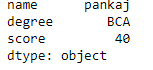#### Accessing a Dataframe with a boolean index using .ix[]

In order to access a dataframe using .ix[], we have to pass boolean value (True or False) and integer value to .ix[] function because as we know that .ix[] function is a hybrid of .loc[] and .iloc[] function.
Code #1:

## Python3

 `# importing pandas as pd` `import` `pandas as pd` ` `  `# dictionary of lists` `dict` `=` `{``'name'``:[``"aparna"``, ``"pankaj"``, ``"sudhir"``, ``"Geeku"``],` `        ``'degree'``: [``"MBA"``, ``"BCA"``, ``"M.Tech"``, ``"MBA"``],` `        ``'score'``:[``90``, ``40``, ``80``, ``98``]}`   `# creating a dataframe with boolean index` `df ``=` `pd.DataFrame(``dict``, index ``=` `[``True``, ``False``, ``True``, ``False``])` ` `    `# accessing a dataframe using .ix[] function` `print``(df.ix[``True``])`

Output: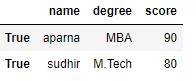Code #2:

## Python

 `# importing pandas as pd` `import` `pandas as pd` ` `  `# dictionary of lists` `dict` `=` `{``'name'``:[``"aparna"``, ``"pankaj"``, ``"sudhir"``, ``"Geeku"``],` `        ``'degree'``: [``"MBA"``, ``"BCA"``, ``"M.Tech"``, ``"MBA"``],` `        ``'score'``:[``90``, ``40``, ``80``, ``98``]}`   `# creating a dataframe with boolean index ` `df ``=` `pd.DataFrame(``dict``, index ``=` `[``True``, ``False``, ``True``, ``False``])` ` `    `# accessing a dataframe using .ix[] function` `print``(df.ix[``1``])`

Output: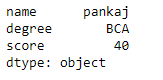Applying a boolean mask to a dataframe :
In a dataframe we can apply a boolean mask in order to do that we, can use __getitems__ or [] accessor. We can apply a boolean mask by giving list of True and False of the same length as contain in a dataframe. When we apply a boolean mask it will print only that dataframe in which we pass a boolean value True. To download “nba1.1” CSV file click here.
Code #1:

## Python3

 `# importing pandas as pd` `import` `pandas as pd` ` `  `# dictionary of lists` `dict` `=` `{``'name'``:[``"aparna"``, ``"pankaj"``, ``"sudhir"``, ``"Geeku"``],` `        ``'degree'``: [``"MBA"``, ``"BCA"``, ``"M.Tech"``, ``"MBA"``],` `        ``'score'``:[``90``, ``40``, ``80``, ``98``]}` ` `  `df ``=` `pd.DataFrame(``dict``, index ``=` `[``0``, ``1``, ``2``, ``3``])` ` `      `print``(df[[``True``, ``False``, ``True``, ``False``]])`

Output: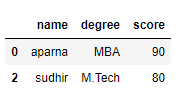Code #2:

## Python3

 `# importing pandas package` `import` `pandas as pd` ` `  `# making data frame from csv file` `data ``=` `pd.read_csv(``"nba1.1.csv"``)` ` `  `df ``=` `pd.DataFrame(data, index ``=` `[``0``, ``1``, ``2``, ``3``, ``4``, ``5``, ``6``,` `                                 ``7``, ``8``, ``9``, ``10``, ``11``, ``12``])`   ` `  `df[[``True``, ``False``, ``True``, ``False``, ``True``,` `    ``False``, ``True``, ``False``, ``True``, ``False``,` `                ``True``, ``False``, ``True``]]`

Output: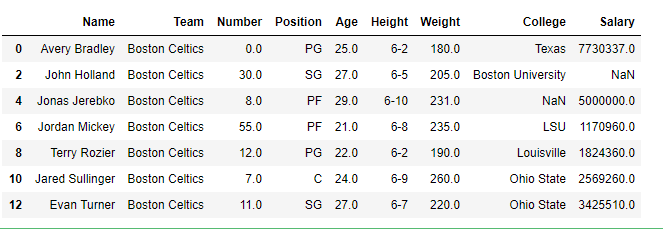Masking data based on column value :
In a dataframe we can filter a data based on a column value in order to filter data, we can apply certain condition on dataframe using different operator like ==, >, <, <=, >=. When we apply these operator on dataframe then it produce a Series of True and False. To download the “nba.csv” CSV, click here.
Code #1:

## Python

 `# importing pandas as pd` `import` `pandas as pd` ` `  `# dictionary of lists` `dict` `=` `{``'name'``:[``"aparna"``, ``"pankaj"``, ``"sudhir"``, ``"Geeku"``],` `        ``'degree'``: [``"BCA"``, ``"BCA"``, ``"M.Tech"``, ``"BCA"``],` `        ``'score'``:[``90``, ``40``, ``80``, ``98``]}`   `# creating a dataframe ` `df ``=` `pd.DataFrame(``dict``)` ` `  `# using a comparison operator for filtering of data` `print``(df[``'degree'``] ``=``=` `'BCA'``)`

Output: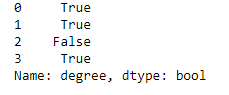Code #2:

## Python

 `# importing pandas package` `import` `pandas as pd` ` `  `# making data frame from csv file` `data ``=` `pd.read_csv(``"nba.csv"``, index_col ``=``"Name"``)` ` `  `# using greater than operator for filtering of data` `print``(data[``'Age'``] > ``25``)`

Output: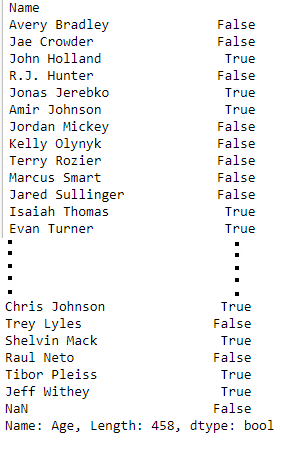Masking data based on index value :
In a dataframe we can filter a data based on a column value in order to filter data, we can create a mask based on the index values using different operator like ==, >, <, etc… . To download “nba1.1” CSV file click here.
Code #1:

## Python3

 `# importing pandas as pd` `import` `pandas as pd` ` `  `# dictionary of lists` `dict` `=` `{``'name'``:[``"aparna"``, ``"pankaj"``, ``"sudhir"``, ``"Geeku"``],` `        ``'degree'``: [``"BCA"``, ``"BCA"``, ``"M.Tech"``, ``"BCA"``],` `        ``'score'``:[``90``, ``40``, ``80``, ``98``]}` ` `    `df ``=` `pd.DataFrame(``dict``, index ``=` `[``0``, ``1``, ``2``, ``3``])`   `mask ``=` `df.index ``=``=` `0`   `print``(df[mask])`

Output: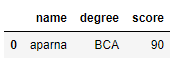Code #2:

## Python3

 `# importing pandas package` `import` `pandas as pd` ` `  `# making data frame from csv file` `data ``=` `pd.read_csv(``"nba1.1.csv"``)`   `# giving a index to a dataframe` `df ``=` `pd.DataFrame(data, index ``=` `[``0``, ``1``, ``2``, ``3``, ``4``, ``5``, ``6``,` `                                 ``7``, ``8``, ``9``, ``10``, ``11``, ``12``])`   `# filtering data on index value` `mask ``=` `df.index > ``7`   `df[mask]`

Output: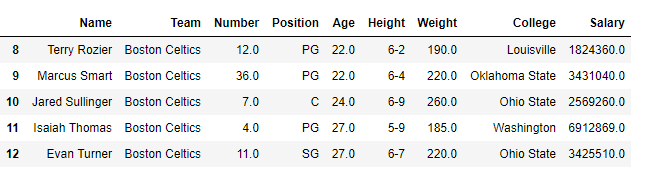Attention geek! Strengthen your foundations with the Python Programming Foundation Course and learn the basics.

To begin with, your interview preparations Enhance your Data Structures concepts with the Python DS Course.

My Personal Notes arrow_drop_up
Recommended Articles
Page :